# Integrated Rate Equations

The integrated rate equations are different for the reactions of different reaction orders. We shall determine these equations only for zero and first order chemical reactions.

## Zero Order Reaction

The rate of the reaction is proportional to zero power of the concentration of reactants.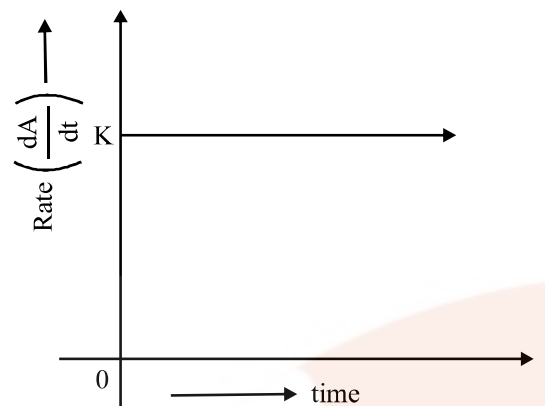As any quantity raised to power zero is unity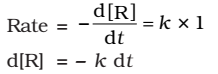Integrating both sides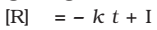Where, I is the constant of integration.

At t = 0, the concentration of the reaction R = [R]0 , where [R]0 is initial concentration of the reaction.

Substituting in equation,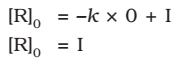Substituting the value of I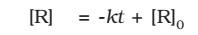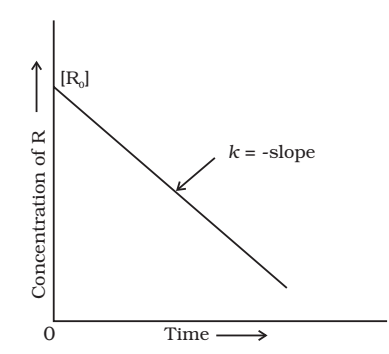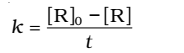Example:

• The decomposition of gaseous ammonia on a hot platinum surface at high pressure.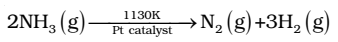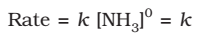• Thermal decomposition of HI on a gold surface.

## First Order Reaction

The rate of the reaction is proportional to the first power of the concentration of the reactant R.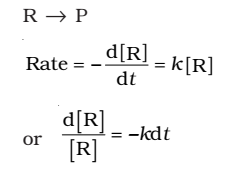Integrating this equation, we get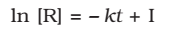Again, I is the constant of integration and its value can be determined easily.

When t= 0, R= [R]0, where [R]0 is the initial concentration of the reactant.

Therefore, equation can be written as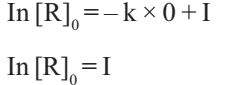Substituting the value of I in equationRearranging this equation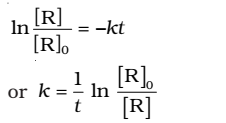At time t1 from above eq.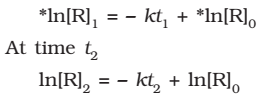Where, [R]1 and [R]2 are the concentrations of the reactants at time t1 and t2 respectively.

Subtracting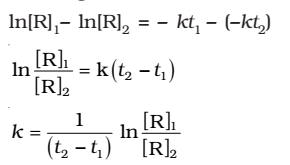Comparing equation (2) with y = mx +c, if we plot In[R] against t, we get a straight line with slope = -k and intercept equal to ln[R]0The first order rate equation (3) can also be written in the form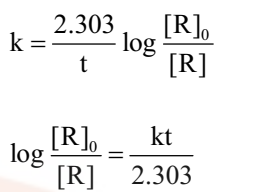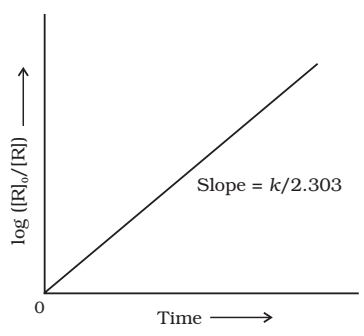Example:

• Hydrogenation of ethane,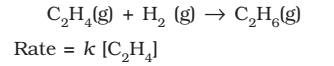• Decomposition of N2O5 and N2O